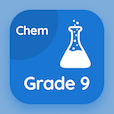Class 9 Online Courses

Grade 9 Chemistry MCQs PDF - Topics

# Atomic and Mass Number MCQ Quiz Online

Learn Atomic and mass number Multiple Choice Questions (MCQ) to practice atomic and mass number quiz answers PDF to study online 9th grade chemistry course. Fundamentals of Chemistry Multiple Choice Questions and Answers (MCQs), Atomic and Mass Number quiz questions PDF for secondary school graduation certificate. "Atomic and Mass Number MCQ" PDF eBook: branches of chemistry, empirical and molecular formulas, ions and free radicals, elements compounds and mixtures test prep for distance learning.

"The mass number of an atom is" Multiple Choice Questions (MCQ) on atomic and mass number with choices number of protons only, number of neutrons only, number of electrons, and number of protons and neutrons for secondary school graduation certificate. Study fundamentals of chemistry quiz questions for online certificate programs for online degree programs.

## MCQs on Atomic and Mass Number

MCQ: The mass number of an atom is

number of protons only
number of neutrons only
number of electrons
number of protons and neutrons

MCQ: The number of protons in the nucleus constitute it's

atomic mass
atomic number
nucleon number
number of neutrons

MCQ: The number of protons and neutrons in the nucleus if the atomic number of an element is 17 and the mass number is 35, is

17 and 18
17 and 19
17 and 20
17 and 21

### More Topics from Grade 9 Chemistry Course